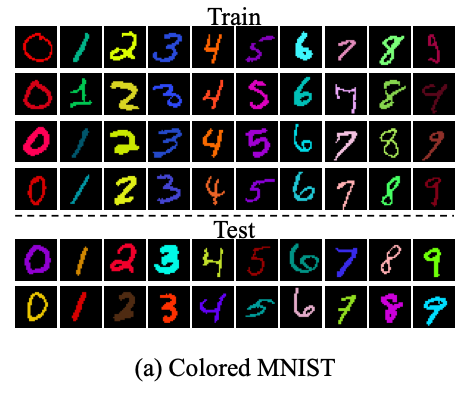### Why Graphs?(Introduction > Why Graphs?)

Graphs are a general language for describing and analyzing entities with relationships/interactions. Many domains have a natural relational structure, that lends themselves to a graph representation:

• Physical roads, bridges, tunnels connecting places. 🚗
• Particles, based on their proximities. ⚛️
• Animals in a food ecosystem. 🕸
• Computer networks. 💻
• Knowledge graphs, scene graphs, code graphs…

### Graph Representations(Graph Representations)

A few different traditional graph representations we can use.

### Counterfactual Generative Networks(Problem setting:)

We have images $$\mathbf{x}$$, and labels $$y$$. In generative modeling, it’s common to assume that each $$\mathbf{x}$$ can be described by some lower-dimensional latent space $$\mathbf{z}$$ (e.g. color, shape, etc.) We’d like this latent representation to be disentangled into several separate, semenatically meaningful factors, so we can control the influence of each on the classifier’s decision. Usually in disentangled modeling, these factors are assumed to be statistically independent– however, in practice this is a poor assumption. One rather contrived example is found in the colored MNIST dataset:We might want to disentangle digit shape from color; however, in the train fold, all examples of the same digit are also the same color. (In the test fold, the colors are randomized.) Given this dataset, a “dumb” neural net might learn to do the simplest thing, which is to count how many pixels are a certain color; if a digit is red, it assumes it must be a 0, if it’s green, a 1, and so on, completely ignoring the digit’s shape.

### What causes changes in distribution?(What causes changes in distribution?)

hypothesis to replace i.i.d. assumption: changes in distribution = consequence of an intervention on one/few causes /mechanisms. So, not identically distributed, but pretty similar, if you’re in the right high-level representation space. (E.g. if you put shaded glasses on, all the pixels change in basic RGB space – but in some high-level semantic space, only one bit changed!)

### 🧐 philosophy

The aim of philosophy, abstractly formulated, is to understand how things in the broadest possible sense of the term hang together in the broadest possible sense of the term.
– Wilfrid Sellars, “Philosophy and the Scientific Image of Man”

Some things we can think about in a philosophical lens…